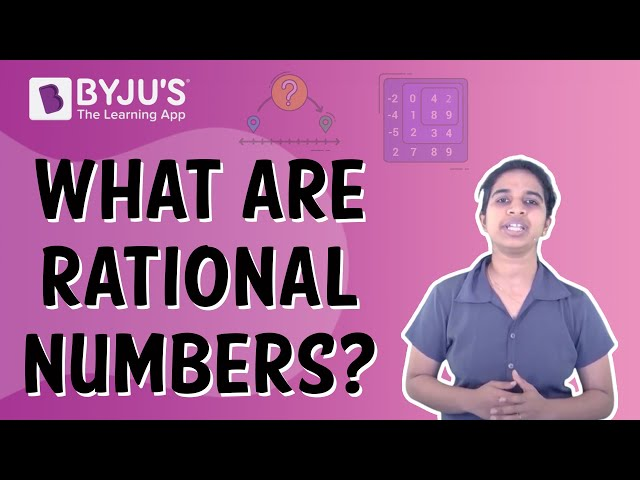# Decimal Expansion Of Rational Numbers

Before going into a representation of the decimal expansion of rational numbers, let us understand what rational numbers are. Any number that can be represented in the form of p/q, such that p and q are integers and q ≠ 0 are known as rational numbers. So when these numbers have been simplified further, they result in decimals. Let us learn how to expand such decimals here.

Examples:

$$\begin{array}{l} 6 , -8.1,\frac{4}{5}\end{array}$$
etc. are all examples of rational numbers.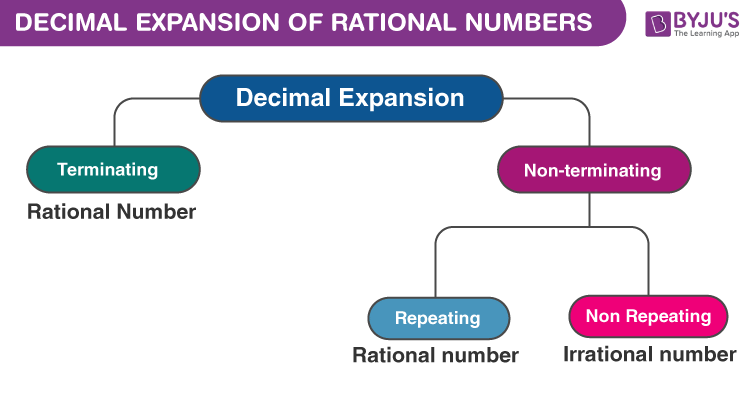## How to Expand Rational Numbers in Decimals?

The real numbers which are recurring or terminating in nature are generally rational numbers.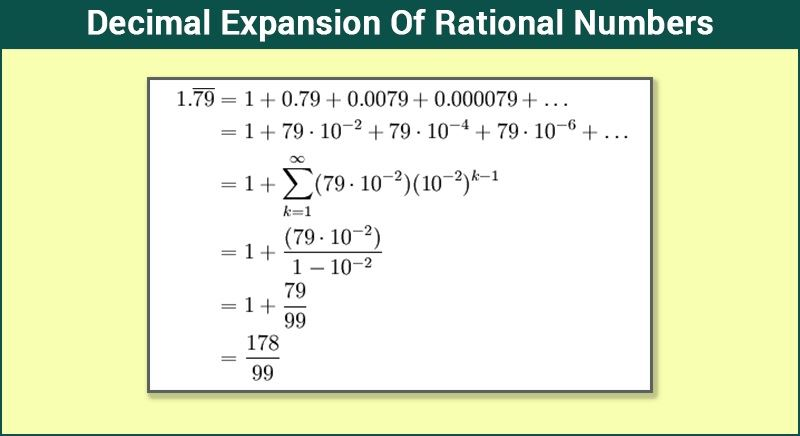For example, consider the number 33.33333……. It is a rational number as it can be represented in the form of 100/3. It can be seen that the decimal part .333…… is the non-terminating repeating part, i .e. it is a recurring decimal number.

Also the terminating decimals such as 0.375, 0.6 etc. which satisfy the condition of being rational (

$$\begin{array}{l}0.375\end{array}$$
=
$$\begin{array}{l}\frac{3}{2^3}\end{array}$$
,
$$\begin{array}{l}0.6\end{array}$$
=
$$\begin{array}{l}\frac{3}{5}\end{array}$$
).

Consider any decimal number. For e.g. 0.567. It can be written as 567/1000 or

$$\begin{array}{l}\frac{567}{10^3}\end{array}$$
. Similarly, the numbers 0.6689,0.032 and .45 can be written as
$$\begin{array}{l}\frac{6689}{10^4}\end{array}$$
,
$$\begin{array}{l}\frac{32}{10^3}\end{array}$$
and
$$\begin{array}{l}\frac{45}{10^2}\end{array}$$
respectively in fractional form.

Thus, it can be seen that any decimal number can be represented as a fraction which has denominator in powers of 10. We know that prime factors of 10 are 2 and 5, it can be concluded that any decimal rational number can be easily represented in the form of

$$\begin{array}{l}\frac{p}{q}\end{array}$$
, such that p and q are integers and the prime factorization of q is of the form
$$\begin{array}{l}2^x~ 5^y\end{array}$$
, where x and y are non-negative integers.

This statement gives rise to a very important theorem.

### Theorems

Theorem 1: If m be any rational number whose decimal expansion is terminating in nature, then m can be expressed in form of

$$\begin{array}{l}\frac{p}{q}\end{array}$$
, where p and q are co-primes and the prime factorization of q is of the form
$$\begin{array}{l}2^x~ 5^y\end{array}$$
, where x and y are non-negative integers.

The converse of this theorem is also true and it can be stated as follows:

Theorem 2: If m is a rational number, which can be represented as the ratio of two integers i.e.

$$\begin{array}{l}\frac{p}{q}\end{array}$$
and the prime factorization of q takes the form
$$\begin{array}{l}2^x~ 5^y\end{array}$$
, where x and y are non-negative integers then, then it can be said that m has a decimal expansion which is terminating.

Consider the following examples:

1. $$\begin{array}{l}\frac{7}{8}\end{array}$$
=
$$\begin{array}{l}\frac{7}{2^3}\end{array}$$
=
$$\begin{array}{l}\frac{7~×~5^3}{2^3~×~5^3}\end{array}$$
=
$$\begin{array}{l}\frac{875}{10^3}\end{array}$$
2. $$\begin{array}{l}\frac{3}{80}\end{array}$$
=
$$\begin{array}{l}\frac{3}{2^4~×~5}\end{array}$$
=
$$\begin{array}{l}\frac{3~×~5^3}{2^4~×~5^4}\end{array}$$
=
$$\begin{array}{l}\frac{375}{10^4}\end{array}$$

Moving on, to decimal expansion of rational numbers which are recurring, the following theorem can be stated:

Theorem 3: If m is a rational number, which can be represented as the ratio of two integers i.e.

$$\begin{array}{l}\frac{p}{q}\end{array}$$
and the prime factorization of q does not takes the form
$$\begin{array}{l}2^x~ 5^y\end{array}$$
, where x and y are non-negative integers. Then, it can be said that m has a decimal expansion which is non-terminating repeating (recurring).

Consider the following examples:

1. $$\begin{array}{l}\frac{1}{6}\end{array}$$
=
$$\begin{array}{l}0.1666….\end{array}$$
=
$$\begin{array}{l}0.1\overline{6}\end{array}$$
2. $$\begin{array}{l}\frac{7}{12}\end{array}$$
=
$$\begin{array}{l}0.58333…\end{array}$$
=
$$\begin{array}{l}0.58\overline{3} \end{array}$$
3. $$\begin{array}{l}\frac{9}{11}\end{array}$$
=
$$\begin{array}{l}0.8181…\end{array}$$
=
$$\begin{array}{l}0.\overline{81}\end{array}$$

### Rational Number to decimal Examples

Case 1: Remainder equal to zero

Example: Find the decimal expansion of 3/6.Here, the quotient is 0.5 and the remainder is 0. Rational number 3/6 results in a terminating decimal.

Case 2: Remainder not equal to zero

Example: Express 5/13 in decimal form.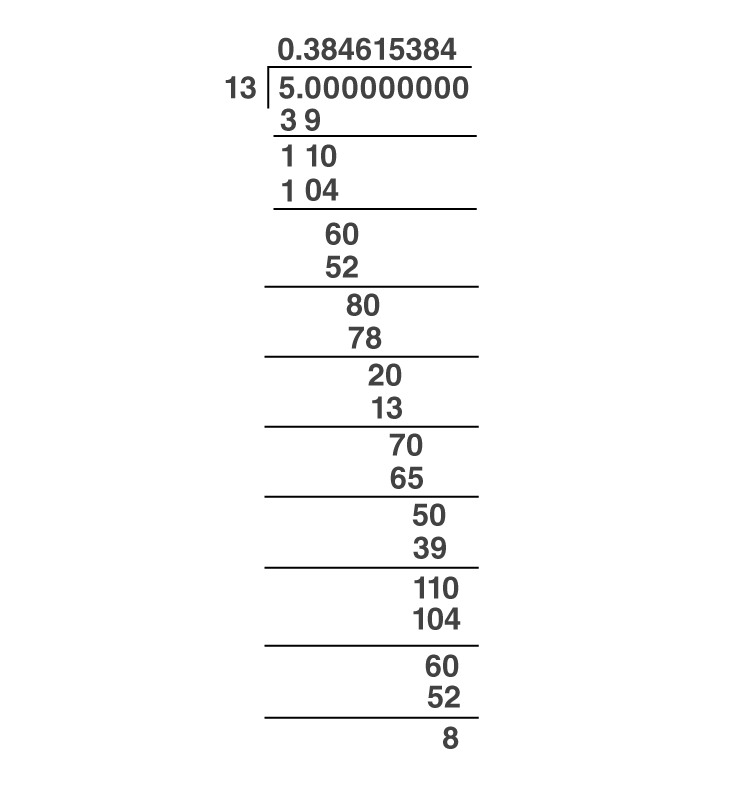Here, the quotient is 0.384615384 and the remainder is not zero. Notice that the number…384 after the decimal is repeating. Hence, 5/13 gives us a non-terminating recurring decimal expansion. And this can be written as 5/13=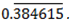A rational number gives either terminating or non-terminating recurring decimal expansion. Thus, we can say that a number whose decimal expansion is terminating or non-terminating recurring is rational.

## Video Lesson on Rational Numbers